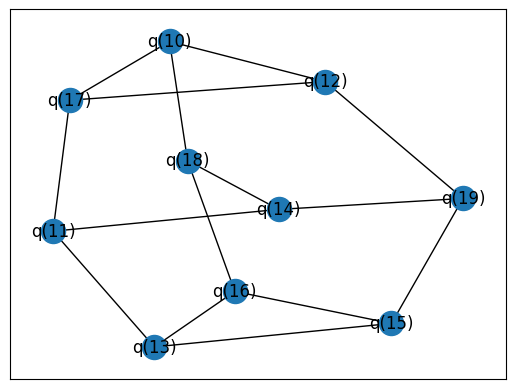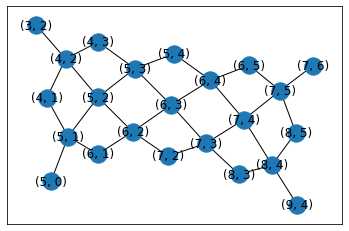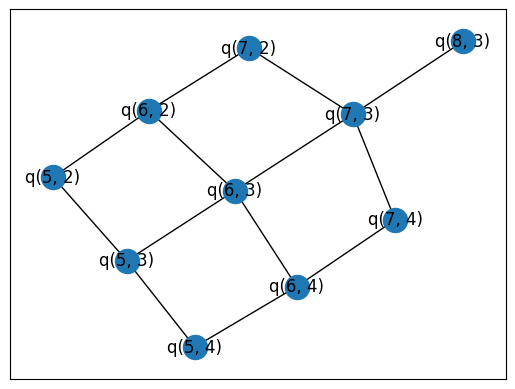# Routing with t|ket>

Wrap tket's compilation unit framework to keep track of qubit mappings and work with generic devices.

## Setup

Install the ReCirq package:

``````try:
import recirq
except ImportError:
!pip install -q git+https://github.com/quantumlib/ReCirq sympy~=1.6
``````

Now import Cirq, ReCirq and the module dependencies:

``````import cirq
import recirq
import networkx as nx
from cirq.contrib.svg import SVGCircuit
import numpy as np
``````
``````from pytket.predicates import CompilationUnit, ConnectivityPredicate
from pytket.passes import SequencePass, RoutingPass, DecomposeSwapsToCXs
from pytket.routing import GraphPlacement
``````

### Example circuit

We'll route a 3-regular circuit to Sycamore23. To try to clear up some of the confusion about which indices are which, we'll construct the initial circuit with `LineQubits` 10 through 19 which should be thought of as "logical indices".

``````from recirq.qaoa.problem_circuits import get_generic_qaoa_circuit
from recirq.qaoa.gates_and_compilation import compile_problem_unitary_to_arbitrary_zz, \
compile_driver_unitary_to_rx

problem_graph = nx.random_regular_graph(d=3, n=10)
nx.set_edge_attributes(problem_graph, values=1, name='weight')
circuit_qubits = cirq.LineQubit.range(10, 20)
gammas = np.random.randn(2)
betas = np.random.randn(2)
circuit = get_generic_qaoa_circuit(
problem_graph=problem_graph,
qubits=circuit_qubits,
gammas=gammas,
betas=betas)
circuit = compile_problem_unitary_to_arbitrary_zz(circuit)
circuit = compile_driver_unitary_to_rx(circuit)
SVGCircuit(circuit)
``````
```findfont: Font family ['Arial'] not found. Falling back to DejaVu Sans.
```### "Route" this circuit

Let's look at the "connectivity graph" of the circuit vs. that of the device

``````import cirq.contrib.routing as ccr

uncompiled_c_graph = ccr.get_circuit_connectivity(circuit)
nx.draw_networkx(uncompiled_c_graph)
````````````import cirq.google as cg

dev_graph = ccr.gridqubits_to_graph_device(cg.Sycamore23.qubits)
nx.draw_networkx(dev_graph)
``````
```/home/kbuilder/.local/lib/python3.7/site-packages/ipykernel_launcher.py:1: DeprecationWarning: cirq.google was used but is deprecated.
it will be removed in cirq v0.14.

"""Entry point for launching an IPython kernel.
`````````# alias for the device. If this notebook were wrapped
# in a function, `circuit` and `device` would be the arguments
device = cg.Sycamore23
``````

### Convert to pytket `Device`

The provided function doesn't work with `SerializableDevice`. We use existing functionality to turn Devices into graphs to provide a more robust solution.

``````import pytket
from pytket.circuit import Node
from recirq.qaoa.placement import _device_to_tket_device

tk_circuit = pytket.extensions.cirq.cirq_to_tk(circuit)
tk_device = _device_to_tket_device(device)
``````
``````tk_circuit.qubits
``````
```[q, q, q, q, q, q, q, q, q, q]
```
``````tk_device.coupling
``````
```[(grid[4, 2], grid[3, 2]),
(grid[4, 2], grid[4, 1]),
(grid[4, 2], grid[4, 3]),
(grid[4, 2], grid[5, 2]),
(grid[4, 1], grid[5, 1]),
(grid[4, 3], grid[5, 3]),
(grid[5, 2], grid[6, 2]),
(grid[5, 2], grid[5, 3]),
(grid[5, 2], grid[5, 1]),
(grid[5, 4], grid[5, 3]),
(grid[5, 4], grid[6, 4]),
(grid[5, 3], grid[6, 3]),
(grid[6, 4], grid[6, 5]),
(grid[6, 4], grid[7, 4]),
(grid[6, 4], grid[6, 3]),
(grid[5, 0], grid[5, 1]),
(grid[5, 1], grid[6, 1]),
(grid[6, 1], grid[6, 2]),
(grid[6, 2], grid[7, 2]),
(grid[6, 2], grid[6, 3]),
(grid[6, 5], grid[7, 5]),
(grid[7, 5], grid[8, 5]),
(grid[7, 5], grid[7, 4]),
(grid[7, 5], grid[7, 6]),
(grid[7, 2], grid[7, 3]),
(grid[6, 3], grid[7, 3]),
(grid[8, 5], grid[8, 4]),
(grid[8, 4], grid[7, 4]),
(grid[8, 4], grid[8, 3]),
(grid[8, 4], grid[9, 4]),
(grid[7, 4], grid[7, 3]),
(grid[7, 3], grid[8, 3])]
```

### Placement and routing pass

``````from pytket.predicates import CompilationUnit, ConnectivityPredicate
from pytket.passes import SequencePass, RoutingPass, DecomposeSwapsToCXs, PlacementPass
from pytket.routing import GraphPlacement
``````
``````unit = CompilationUnit(tk_circuit, [ConnectivityPredicate(tk_device)])
passes = SequencePass([
PlacementPass(GraphPlacement(tk_device)),
RoutingPass(tk_device)])
passes.apply(unit)
valid = unit.check_all_predicates()
assert valid
``````

### The initial mapping

This maps from logical LineQubits to "physical" GridQubits

``````unit.initial_map
``````
```{q: grid[4, 3],
q: grid[5, 4],
q: grid[5, 1],
q: grid[6, 4],
q: grid[4, 1],
q: grid[4, 2],
q: grid[5, 0],
q: grid[5, 2],
q: grid[5, 3],
q: grid[3, 2]}
```

### Bookkept initial mapping

We "decode" our tket conventions back into Cirq idioms.

``````def tk_to_cirq_qubit(tk):
ind = tk.index
return cirq.LineQubit(ind) if len(ind) == 1 else cirq.GridQubit(*ind)

initial_map = {tk_to_cirq_qubit(n1): tk_to_cirq_qubit(n2) for n1, n2 in unit.initial_map.items()}
initial_map
``````
```{cirq.LineQubit(10): cirq.GridQubit(4, 3),
cirq.LineQubit(11): cirq.GridQubit(5, 4),
cirq.LineQubit(12): cirq.GridQubit(5, 1),
cirq.LineQubit(13): cirq.GridQubit(6, 4),
cirq.LineQubit(14): cirq.GridQubit(4, 1),
cirq.LineQubit(15): cirq.GridQubit(4, 2),
cirq.LineQubit(16): cirq.GridQubit(5, 0),
cirq.LineQubit(17): cirq.GridQubit(5, 2),
cirq.LineQubit(18): cirq.GridQubit(5, 3),
cirq.LineQubit(19): cirq.GridQubit(3, 2)}
```

### The final mapping

This maps from logical LineQubits to final GridQubits

``````unit.final_map
``````
```{q: grid[5, 3],
q: grid[6, 4],
q: grid[5, 0],
q: grid[5, 2],
q: grid[4, 2],
q: grid[3, 2],
q: grid[5, 1],
q: grid[4, 1],
q: grid[5, 4],
q: grid[4, 3]}
```
``````final_map = {tk_to_cirq_qubit(n1): tk_to_cirq_qubit(n2)
for n1, n2 in unit.final_map.items()}
final_map
``````
```{cirq.LineQubit(10): cirq.GridQubit(5, 3),
cirq.LineQubit(11): cirq.GridQubit(6, 4),
cirq.LineQubit(12): cirq.GridQubit(5, 0),
cirq.LineQubit(13): cirq.GridQubit(5, 2),
cirq.LineQubit(14): cirq.GridQubit(4, 2),
cirq.LineQubit(15): cirq.GridQubit(3, 2),
cirq.LineQubit(16): cirq.GridQubit(5, 1),
cirq.LineQubit(17): cirq.GridQubit(4, 1),
cirq.LineQubit(18): cirq.GridQubit(5, 4),
cirq.LineQubit(19): cirq.GridQubit(4, 3)}
```

### The compilation unit applies the mapping

So our circuit qubits are now GridQubits

``````unit.circuit.qubits
``````
```[grid[3, 2],
grid[4, 1],
grid[4, 2],
grid[4, 3],
grid[5, 0],
grid[5, 1],
grid[5, 2],
grid[5, 3],
grid[5, 4],
grid[6, 4]]
```

### Convert the circuit back to Cirq

``````routed_circuit = pytket.extensions.cirq.tk_to_cirq(unit.circuit)
SVGCircuit(routed_circuit)
``````### Now it's nice and compiled

``````routed_c_graph = ccr.get_circuit_connectivity(routed_circuit)
nx.draw_networkx(routed_c_graph)
``````### Check that circuits are equivalent

``````for _, op, _ in routed_circuit.findall_operations_with_gate_type(cirq.TwoQubitGate):
a, b = op.qubits
``````
``````import cirq.contrib.acquaintance as cca
def permute_gate(qubits, permutation):
return cca.LinearPermutationGate(
num_qubits=len(qubits),
permutation={i: permutation[i] for i in range(len(permutation))}
).on(*qubits)

final_to_initial_map = {final_map[cq]: initial_map[cq]
for cq in circuit_qubits}
initial_qubits = [initial_map[cq] for cq in circuit_qubits]
final_permutation = [initial_qubits.index(final_to_initial_map[q])
for q in initial_qubits]
rcircuit_with_perm = routed_circuit.copy()
rcircuit_with_perm.append(permute_gate(initial_qubits, final_permutation))
expected = circuit.unitary(qubit_order=cirq.QubitOrder.explicit(circuit_qubits))
actual = rcircuit_with_perm.unitary(qubit_order=cirq.QubitOrder.explicit(initial_qubits))
cirq.testing.assert_allclose_up_to_global_phase(expected, actual, atol=1e-8)
``````
[{ "type": "thumb-down", "id": "missingTheInformationINeed", "label":"Missing the information I need" },{ "type": "thumb-down", "id": "tooComplicatedTooManySteps", "label":"Too complicated / too many steps" },{ "type": "thumb-down", "id": "outOfDate", "label":"Out of date" },{ "type": "thumb-down", "id": "samplesCodeIssue", "label":"Samples / code issue" },{ "type": "thumb-down", "id": "otherDown", "label":"Other" }]
[{ "type": "thumb-up", "id": "easyToUnderstand", "label":"Easy to understand" },{ "type": "thumb-up", "id": "solvedMyProblem", "label":"Solved my problem" },{ "type": "thumb-up", "id": "otherUp", "label":"Other" }]